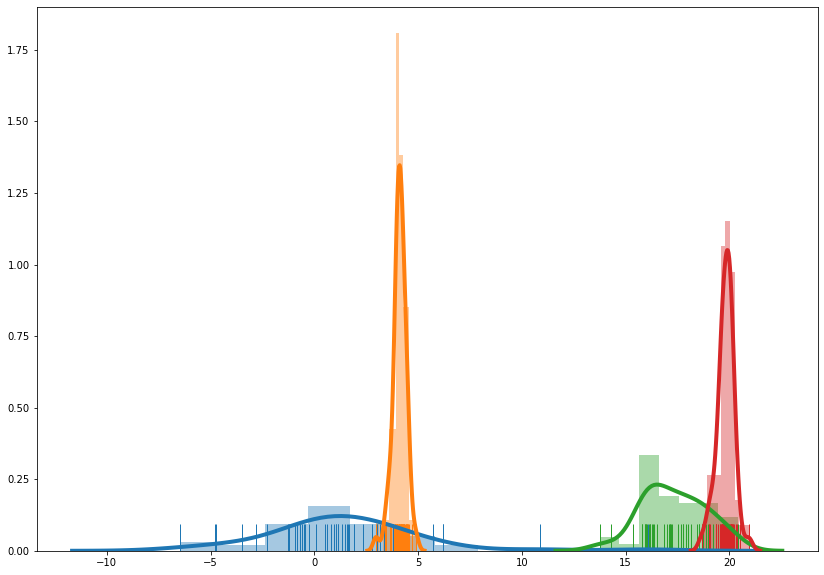In :
import numpy as np
import pandas as pd
import matplotlib.pyplot as plt
import seaborn as sns


# Variational Inference¶

Inference and learning often involve intractable integrals.

Prime examples include bayesian inference

$$p(y | \mathbf{x}, \mathcal{D})=\int p(y, \mathbf{w} | \mathbf{x}, \mathcal{D}) \mathrm{d} \mathbf{w}=\int p(y | \mathbf{x}, \mathbf{w}) p(\mathbf{w} | \mathcal{D}) \mathrm{d} \mathbf{w}$$

or marginalization of unseen variables

$$L(\boldsymbol{\theta})=p(\mathcal{D} ; \boldsymbol{\theta})=\int_{\mathbf{u}} p(\mathbf{u}, \mathcal{D} ; \boldsymbol{\theta}) \mathrm{d} \mathbf{u}$$

There are two possible methods to approximate such integrals:

• Monte Carlo estimate through sampling
• Variational approach

In this notebook I will focus on this second approach.

## Kullback-Leibler divergence¶

The KL divergence is a fundamental concept in variational inference and consequently for variational autoencoders. The KL divergence between two distributions $p$ and $q$ is:

$$\mathrm{KL}(p \| q)=\int p(\mathbf{x}) \,\log \left(\frac{p(\mathbf{x})}{q(\mathbf{x})} \right)\mathrm{d} \mathbf{x}=\mathbb{E}_{p(\mathbf{x})}\left[\log \frac{p(\mathbf{x})}{q(\mathbf{x})}\right]$$

Properties of KL divergence:

• $\mathrm{KL}(p \| q)=0$ iff $p = q$
• $\mathrm{KL}(p \| q)\neq \mathrm{KL}(q \| p)$ (non-commutative)
• $\mathrm{KL}(p \| q)\geq0$ (always non-negative)

## Variational principle¶

Given a joint distribution $p(\mathbf{x}, \mathbf{y})$, the Variational principle states that we can formulate inference tasks such as marginalization $p(\mathbf{x})=\int p(\mathbf{x}, \mathbf{y}) \mathrm{d} \mathbf{y}$, and conditioning $p(\mathbf{y} | \mathbf{x})$, as optimization problems.

Specifically, the maximisation of variational free energy

$$\mathcal{F}(\mathbf{x}, q)=\mathbb{E}_{q(\mathbf{y})}\left[\log \frac{p(\mathbf{x}, \mathbf{y})}{q(\mathbf{y})}\right]$$

• $\log p(\mathbf{x})=\max _{q(\mathbf{y})} \mathcal{F}(\mathbf{x}, q)$
• $p(\mathbf{y} | \mathbf{x})=\operatorname{argmax}_{q(\mathbf{y})} \mathcal{F}(\mathbf{x}, q)$

By separating the joint distribution $p$ in the formulation of the free energy, we find that $$\log p\left(\mathbf{x}\right)=\mathrm{KL}\left(q(\mathbf{y}) \| p\left(\mathbf{y} | \mathbf{x}\right)\right)+\mathcal{F}\left(\mathbf{x}, q\right)=\mathrm{const}$$

Meaning that maximising the variational free energy is equivalent to minimising the KL divergence $\mathrm{KL}(q \| p)$

Since the KL-divergence is always non-negative, $\mathcal{F}$ is also referred to as the Evidence Lower Bound (ELBO), since it provides a lower bound for the marginal likelihood. $$\log p\left(\mathbf{x}\right)\geq\mathcal{F}\left(\mathbf{x}, q\right)$$

In variational inference the $q$ distribution involved in the ELBO is parametrised as $q(\mathbf{y}; \mathbf{\theta})$, and the parameters are optimised to push the ELBO as high as possible.

## Example implementation: variational inference in 1D¶

In :
# Generate the data: let us consider a mixture of laplacian distributions
SEED = 2314
N = 200 # number of points
K = 4 # distributions in the mixture
np.random.seed(SEED)
source_distr = np.random.randint(0, K, N)
data = np.zeros(N)

fig, ax = plt.subplots(figsize=(14, 10))
for i in range(K):
mean = 20* np.random.random()
scale = 3 * np.random.random()

idxs = np.where(source_distr==i)
laplacian_data = np.random.laplace(mean, scale, len(idxs))
data[idxs] = laplacian_data
sns.distplot(laplacian_data, bins="auto", kde=True, rug=True, kde_kws={"linewidth": 4}, ax=ax)In :
# Fitting a gaussian model

In [ ]: